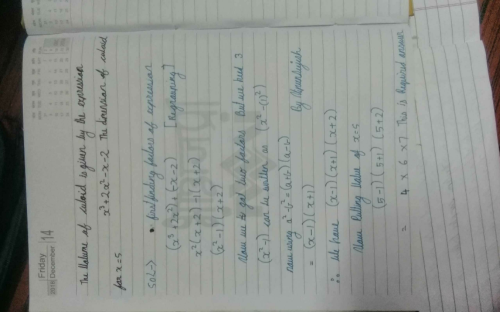Courses

# Can you explain the answer of this question below:The volume of cuboid is given by the expression x3+2x2-x-2. The dimension of the cuboid for x = 5 is:​A:4×6×7B:2×3×4C:5×6×7D:6×7×8The answer is a. Related Test: Test: Factorisation

## Class 9 Question

By Bhumika Chauhan · Sep 12, 2018 ·Class 9
Nipun S answered Sep 12, 2018Abhishek Roy answered Sep 19, 2020
A

Arzoo Sharma answered Sep 02, 2018
There is problem in q I think you should also give the ratio of length, breadth and height. ( upvote if agree )

Archish Kansal answered May 21, 2018
For x=5, Volume is: 5^3 + 2(5^2) - 5 - 2 = 125 + 50 - 5 - 2 = 168
As, volume of cuboid = l x b x h, therefore, dimensions = 4 x 6 x 7.

Achal Chinavalkar answered Sep 12, 2018
X=5
x3 2x2-x-2
= 5^3 2*5^2-5-2
=125 50-7
=118 50
=168

option a ) 4*6*7=168 therefore a is answer